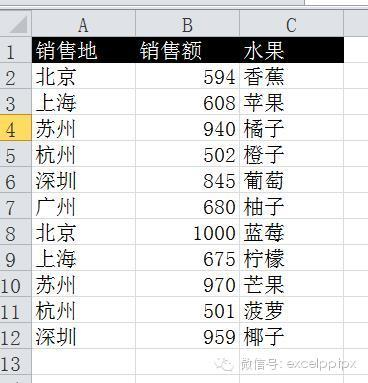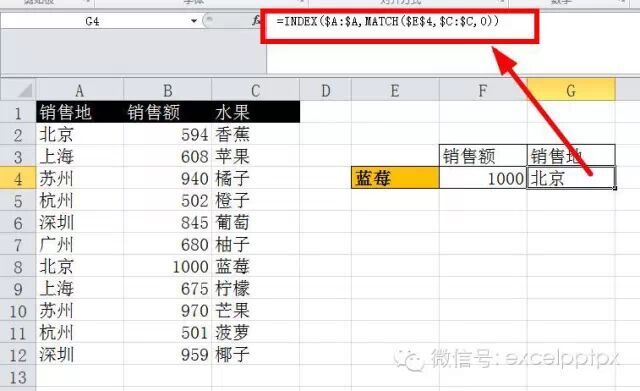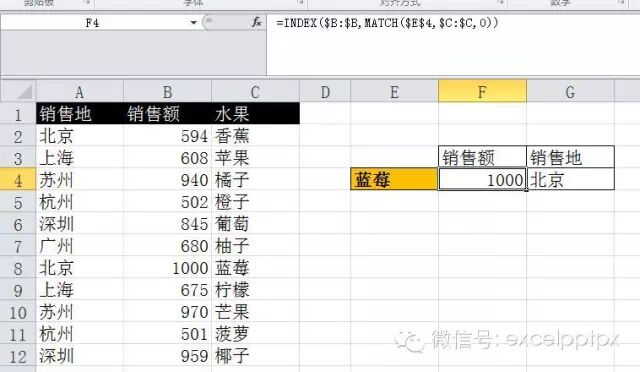Question to say "I can!"

# excel反向查找,不用vlookup，用lookup或index

2014-10-31=INDEX(\$B:\$B,MATCH(\$E\$4,\$C:\$C,0))

=INDEX(\$A:\$A,MATCH(\$E\$4,\$C:\$C,0))

=LOOKUP(1,0/(\$E\$4=\$C\$2:\$C\$12),\$B\$2:\$B\$12)

=LOOKUP(1,0/(\$E\$4=\$C\$2:\$C\$12),\$A\$2:\$A\$12)